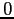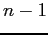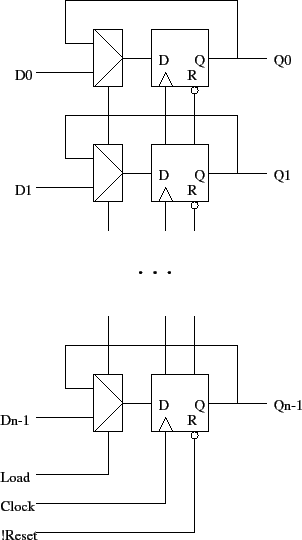# Registers

Tom Kelliher, CS 240

Apr. 20, 2012

### From Last Time

VHDL for sequential circuits.

### Outline

1. Definitions.

2. Parallel registers.

3. VHDL for registers.

Shift registers.

# Definitions

1. Register: A set of flip-flops which have common clock and control signals and load related bits -- a whole word, data on a bus.

Examples: register file, MDR, MAR.

2. Counter: A register which passes through a pre-determined set of states. Usually they count by one fromto.

Example: program counter.

# Parallel Registers

Use a mux approach:Gating the clock will save the muxes, but clock gating is usually a bad idea -- clock skew problems.

1. Really bad: `clockToFF = load and clock`

2. Just as bad: `clockToFF = !load or clock`

Show waveforms and show what skew looks like and why we care.

# VHDL for Registers

Note: The handling of the load signal could be used to implement a synchronous reset.

```-- VHDL for 32 bit parallel load register with asynchronous low
-- reset.

library ieee;
use ieee.std_logic_1164.all;

entity parallel_register is

port (
clock, load, reset_n : in  std_logic;
d                    : in  std_logic_vector (31 downto 0);
q                    : out std_logic_vector (31 downto 0));

end parallel_register;

architecture dataflow of parallel_register is

signal state : std_logic_vector (31 downto 0);

begin  -- dataflow

q <= state;                           -- Output process.

state_reg : process (clock, reset_n)
begin  -- process state
if (reset_n = '0') then             -- asynchronous reset (active low)
state <= X"00000000";
elsif (clock'event and clock = '1') then  -- rising clock edge
state <= d;
else
state <= state;
end if;
end if;
end process state_reg;

end dataflow;
```

Thomas P. Kelliher 2012-04-24
Tom Kelliher